# Pairs of Angles

Pairs of Angles
Go back to  'Angles'

Suppose that a ray OC stands on a line AB, as shown below: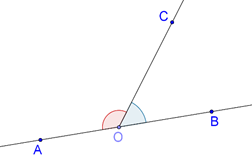We have a pair of adjacent angles, and this pair is a linear pair, which means that the sum of the (measures of the) two angles will be 1800.  This is true in general, and we formalize it as an axiom.

Axiom: If a ray stands on a line, the sum of the pair of adjacent angles is 1800.

Will the converse of this statement be true? That is, if the sum of a pair of adjacent angles is 1800, will the non-common arms of the two angles form a line? The answer is yes, and we formalize it as an axiom as well.

Axiom: If the sum of two adjacent angles is 1800,  then the non-common arms of the angles form a line.

These two axioms can be grouped together as the Linear Pair Axiom.

Suppose that two lines AB and CD intersect at O, as shown below: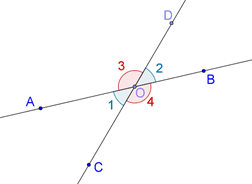Observe how we have marked the various angles using numbers. Note that $$\angle 1$$ and $$\angle 2$$ form a pair of vertically opposite angles. Similarly, $$\angle 3$$ and $$\angle 4$$ form another pair of vertically opposite angles. What is the relation between vertically opposite angles? Intuitively, it should be obvious that they are equal. Let us prove this fact rigorously.

Theorem: Vertically opposite angles are equal.

Proof: Note from the figure that:

$$\angle 1{\rm{ }} + \angle 3{\rm{ }} = {\rm{ }}{180^0}$$          (Linear pair on line COD)

$$\angle 2{\rm{ }} + \angle 3{\rm{ }} = {\rm{ }}{180^0}$$          (Linear pair on line AOB)

Thus,

$$\angle 1{\rm{ }} + \angle 3{\rm{ }} = \angle 2{\rm{ }} + \angle 3$$

è$$\angle 1{\rm{ }} = \angle 2$$

Similarly, we can show that $$\angle 3{\rm{ }} = \angle 4$$

Example 1: Two lines AB and CD intersect at O, forming the angles as shown below: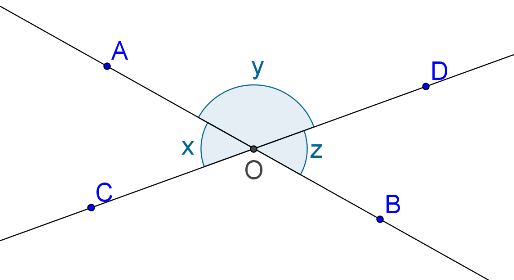If x:y = 3:7, what is the value of z?

Solution: Note that x and y form a linear pair, while x and z are vertically opposite angles. Since x:y = 3:7, we let x = 3k and y = 7k. Now, we have:

$$\angle x{\rm{ }} + \angle y{\rm{ }} = {\rm{ }}{180^0}$$

è $$3k{\rm{ }} + {\rm{ }}7k{\rm{ }} = {\rm{ }}180$$

è $$10k{\rm{ }} = {\rm{ }}180$$

è k = 18

Thus, $$\angle x{\rm{ }} = {\rm{ }}{54^0}$$ and $$\angle y{\rm{ }} = {\rm{ }}{126^0}$$. This means that $$\angle z$$ is equal to 540 (the same as $$\angle x$$, since the two are vertically opposite angles).

Example 2: If two adjacent angles form a linear pair, show that their angle bisectors are perpendicular.

Solution: Consider the following figure, in which $$\angle AOC$$ and $$\angle BOC$$ are adjacent angles which form a linear pair: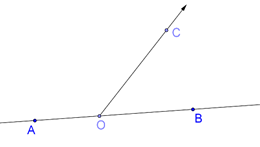Now, we draw the angle bisectors OX and OY of these two angles (an angle bisector of an angle is a line which divides the angle into two equal halves):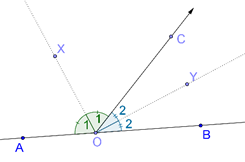We have marked $$\angle AOX {\rm{ }} = \angle COX {\rm{ }} = \angle 1$$ and $$\angle BOY{\rm{ }} = \angle COY{\rm{ }} = \angle 2$$. Since AOB is a straight line, ∠AOB = 1800, and so:

$$\angle 1{\rm{ }} + \angle 1{\rm{ }} + \angle 2{\rm{ }} + \angle 2{\rm{ }} = {\rm{ }}{180^0}$$

è $$2(\angle 1{\rm{ }} + \angle 2){\rm{ }} = {\rm{ }}{180^0}$$

è $$\angle 1{\rm{ }} + \angle 2{\rm{ }} = {\rm{ }}{90^0}$$

è $$\angle XOY{\rm{ }} = {\rm{ }}{90^0}$$

This shows that OX ⊥ OY.

Example 3: Five rays originate from a point O, as shown below: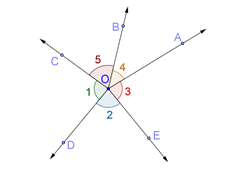Five angles are formed, which have been marked as shown. What is the sum of these angles?

Solution: To solve this problem, we draw a straight line through O (any straight line will do):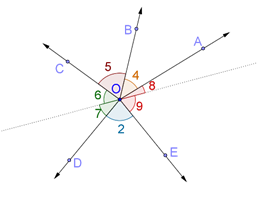Note that $$\angle 1$$ and $$\angle 3$$ have each been divided into two angles, which have been marked. Now, we have:

$\angle 6{\rm{ }} + \angle 5{\rm{ }} + \angle 4{\rm{ }} + \angle 8{\rm{ }} = {\rm{ }}{180^0}$

$\angle 7{\rm{ }} + \angle 2{\rm{ }} + \angle 9{\rm{ }} = {\rm{ }}{180^0}$

If we add the two relations above, $$\angle 6$$ and $$\angle 7$$ add up to $$\angle 1$$, and $$\angle 8$$ and $$\angle 9$$ add up to $$\angle 3$$. Thus, on the left side, we will obtain $$\angle 1{\rm{ }} + \angle 2{\rm{ }} + \angle 3{\rm{ }} + \angle 4{\rm{ }} + \angle 5$$, and on the right side, we will have 3600. This means that the required sum is 3600.

Note that this answer will be the same regardless of how many rays originate from O. This is because when you draw a line through O, all angles on one side of it sum to 1800, and so do all angles on the other side, so the combined sum is always 3600.

Example 4: In the following figure L1 and L2 are two perpendicular lines which intersect at O. Through O, another straight line L is drawn; some angles thus formed have been marked. If $$x:y{\rm{ }} = {\rm{ }}1:5$$, what is the value of $$\angle z$$?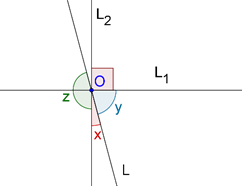Solution: We let x = k and y = 5k. Since the sum of $$\angle x$$ and $$\angle y$$ must be 900, we have:

$$\angle x{\rm{ }} + \angle y{\rm{ }} = {\rm{ }}{90^0}$$

è $$k{\rm{ }} + {\rm{ }}5k{\rm{ }} = {\rm{ }}90$$

è $$6k{\rm{ }} = {\rm{ }}90$$

è $$k{\rm{ }} = {\rm{ }}15$$

Thus, $$\angle x{\rm{ }} = {\rm{ }}{15^0}$$ and $$\angle y{\rm{ }} = {\rm{ }}{75^0}$$. Since $$\angle x$$ and $$\angle z$$ form a linear pair, we have:

$\angle z{\rm{ }} = {\rm{ }}{180^0}--\angle x{\rm{ }} = {\rm{ }}{165^0}$

Geometry
grade 9 | Questions Set 1
Geometry
Geometry